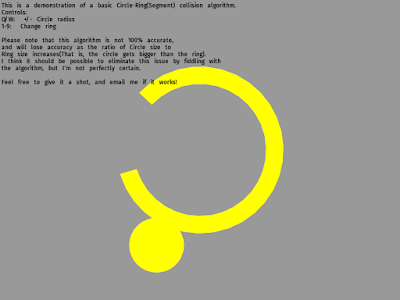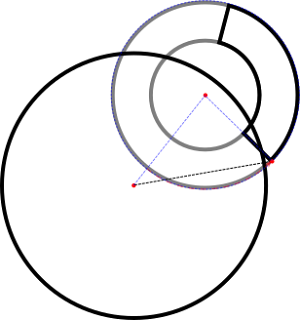4 Hugues Ross - Blog: Collision testing with with segments of a ring
Hugues Ross

## 5/12/15

### Collision testing with with segments of a ring

"I'm having a huge problem getting collision to work in my game. The game involves rotating rings around a planet and protecting it from enemies. My problem is that I can't find a technique that works for this. They are curved and constantly rotating so their height, width, x and y values change all the time. Any ideas??"
Most of the answers given were just suggesting pixel-perfect collision, which seemed like a bit of a cop-out to me. In many cases, pixel-perfect collision is somewhat unnecessary, and slow as well. If it's possible to do without it, I try to avoid pixel-perfect collisions and just go with a math-based check. Thus, I took the post as a bit of a challenge.

### Defining The Problem

Before jumping straight into a solution, we need to know what the problem actually is. First, I should probably clarify what a ring segment is in this case.

Picture a donut, as seen from above. Now, imagine you were to cut a slice out of the donut. The resulting slice is what I refer to as a ring segment. You can also consider it a sort of curved rectangle, as well. If you're more of a visual type, here's a diagram:
Because one of these slices is cut straight from a ring, it can be defined with four values. The first two values, radius and width, represent the original ring. The radius is actually the radius of an invisible circle running through the ring's center. The width then expands from that, giving us the inside of the ring as r - 0.5w, and the outside as r + 0.5w. The other two values are the cut angles. The angles tell us what portion of the ring the segment takes up. If you notice, combining these two sets of values does exactly what I described above: It creates a ring, then cuts out a slice.It might have been simpler just to use inner/outer radius, but I didn't think of that at the time.

Now that we have our ring segment, we can finish the problem. Our goal is simple: Given a circle and a segment, with arbitrary positions/rotations, we must determine if they overlap or not.

### My Solution

As with the Circle-Rectangle collision algorithm, which I can post about if there's any interest in the subject, the goal of my Circle-Ring Segment algorithm is to reduce the second shape to a single 'closest point', then test it against the Circle's radius using the Pythagorean Theorem (A^2+B^2=C^2, perfect for testing the distance between 2 points). Since our ring segment is inherently radial in nature, we can represent the closest point with a magnitude and direction, a.k.a. a vector.

The magnitude is fairly easy to get. All we need is the distance between the radii of the circle and ring. Then, we clamp the magnitude to the inner/outer radius of the ring.

The direction is somewhat trickier, despite sounding simple. If the direction from the radius of the ring to the radius of the circle falls within the angles that form the ring segment, then that is the direction. Otherwise, the direction must be clamped to the closest of the two angles that form the segment. If this sounds familiar, that's because it is--we just did this to get the magnitude. However, doing this with angles can be very tricky. As far as I am aware, the method is as follows:
1. Translate all angles so that they fall between 0 and 360 degrees, or 0 and 2π radians. That way, we can accurately compare their values.
2. Determine if the lower angle has a higher or lower value. The lower angle can actually be larger than the higher angle, when crossing over 0, and it's important to keep this in mind.
3. With the previous information, determine which angle is closest to ours.
The main factor that makes this difficult is the fact that angles effectively 'wrap', returning to 0 once they hit a specified maximum.
Once we have a vector, we can combine it with our ring segments radius to get the closest point. From there, the process is as simple as testing whether the point lies within the circle or not. If it does, the shapes are overlapping.

### The Demo

To test and demonstrate this method, I've constructed a simple demo application. Within it, you can place and size a circle, and see it collide with 9 different preset segments. It should run fine on Windows and Linux computers, and all code is included with the binaries.A bit plain, but it gets the point acrossNotice how the misplaced 'closest point' fails to fall within the circle, resulting in a miss.